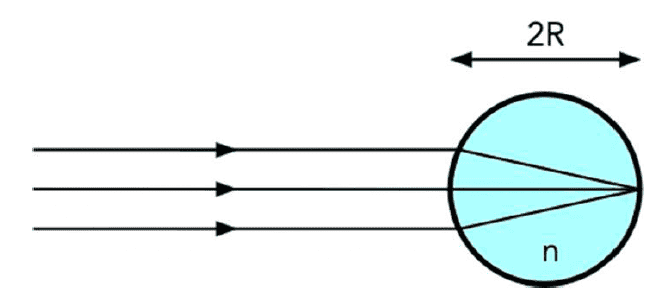# Light incident on a sphere, focused at a distance ##2R##

lorenz0
Homework Statement:
A solid sphere with index of refraction ##n## and radius ##R##, placed in air, is illuminated by a beam of light coming from a source at a great distance, which is focused on the bottom surface of the sphere. Determine the value of ##n##.
Relevant Equations:
##\frac{n_1}{p}+\frac{n_2}{q}=\frac{n_2-n_1}{R}##
I used the equation for the refraction on a spherical surface: ##\frac{n_1}{p}+\frac{n_2}{q}=\frac{n_2-n_1}{R}##, where ##n_1=1## is the index of refraction of air, ##n_2## the index of refraction of the sphere, ##R## is the radius of the glass sphere, ##p## is the object distance which, since the rays are parallel I assumed to be infinite, ##p=\infty##, and ##q## is the image distance, which should be ##q=2R##. Substituting and solving for ##n_2## I get ##n_2=2##.

Does my reasoning make sense? Thanks.

#### Attachments

•refraction.png
10.8 KB · Views: 25